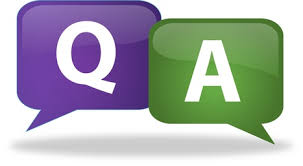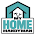# SCC Education

## Rational NumberRational Number
Q.1. Write.
(i) The rational number that does not have a reciprocal.
(ii) The rational numbers that is equal to their reciprocals.
(iii) The rational number that is equal to its negative.
(iv) The additive inverse of a negative number

Answer: (i) 0 (ii) 1 and (–1) (iii) 0 (iv) Negative

Q. 2. Fill in the blanks.
(i) Zero has ________ reciprocal.
(ii) The numbers ________ and ________ are their own reciprocals
(iii) The reciprocal of – 5 is ________.
(iv) Reciprocal of 1/x , where x ≠ 0 is ________.
(v) The product of two rational numbers is always a _______.
(vi) The reciprocal of a positive rational number is ________.
(vii)The number which can be written in the form of p/q , where q≠0, is called _______ number.
(A) Rational (B) Irrational (C) Real (D) Natural

(viii) All rational numbers have multiplicative inverse except _______ .
(A) -1 (B) 1 (C) 0 (D) None

(ix) P and q in p/q form of rational number is ----- ------
(A) primes (B) Co primes (C) Rational (D) Natural

(x) A rational number p/q is said to be in the simplest form if the HCF of p and q is
(a) 2 (b) 1 (c) 0 (d) 3

(xi) Between any two distinct rational numbers there exist (a) Finite rational numbers  (b) Infinite rational numbers (a) No rational number (d) None of the above

(xii) A rational number a/b is greater than c/d if
(a) ad > bc (b) ad < bc (c) ad = bc (d) ad ≠ bc

(xiii) Is zero a rational number (a) Yes (b) No (c) Can‟t say

(xiv) Rational numbers are not closed under
(a) Addition (b) Multiplication (c) Division (d) Subtraction

(xv) If the additive inverse of “b” is “a” then:
(A) axb=1 (B) a=b (C) a+b=0 (D) a-b=0
Answer: (i) No (ii) 1, –1 (iii) -1/5 (iv) x (v) Rational number (vi) positive (vii) Rational number (viii) C (ix) Co primes (x) (b) (xi) (b) (xii) (a) (xiii) (a) (xiv) (c) (xv) (c) 3

. Solve:

1. If you subtract 1/2 from a number and multiply the result by 1/2, you get 1/8. What is the number?

2. Three consecutive integers are such that when they are taken in increasing order and multiplied by 2, 3, and 4 respectively, they add up to 74. Find these numbers.

4. Represent the following rational numbers on the number line

(a) -1/4 (b) −1 1 5 (c) -3 8 5 (d) -7/10 (e) -5/3

5. Find two rational numbers between (i) -2 and 2. (ii) -1 and 0.

6. Insert six rational numbers between (i) -1/3 and -2/3 (ii) ¼ and ½

7. Arrange the following numbers in ascending order: 4 /− 9 , − 5 /12 ; 7/ − 18 ; − 2/ 3

8. Arrange the following numbers in descending order: − 5/6 − 7/12 ; − 13/28 ; 23/−24

9 Represent 4 2 3 on the number line.

10. What number should be added to −7/8to get 4/9?

11. The sum of two rational numbers is −1/2. If one of the numbers is 5/6, find the other.

12. What number should be subtracted from −2/3 to get −1/2?

13. Verify whether the given statement is true or not: (i) (59÷13) ÷ 52 = 59÷(13÷52)

14. Divide the sum of 13/5and−12/7by the product of −31/7and−1/2.

15. The product of two rational numbers is −16/9. If one of the numbers is −4/3, find the other.

16. Find three rational numbers between 4 and 5.

17. Find three rational numbers between 2/3 and 3/4.

18. Find the HCF of 9/10, 12/25, 18/35, 21/40 [Hint: HCF of Fraction= (HCF of Numerators/LCM of denominators=3/1400)

19. After reading 7/9 of a book, 40 pages are left. How many pages are there in the book?

20. A drum full of rice weights 401 6 kg. If the empty drum weights 133 4 kg, find the weight of rice in the drum.

21. Raju earns Rs16000/month. He spends ¼ of his income on food; 3/10 of the remainder on house rent and 5/ 21of the remainder on education of children. How much money is still left with him?22.

Multiple Choice Questions

1. For what value of „a‟ the number -11/a is not a rational number.
( a) -1 (b) 1 (c) 0 (d) 10 [(b) 1]

3. Find the value of. (-9/5) + -(8 /5) ÷ (5/2) x (- 5/4 )
( a) -1 (b) -3 (c) 2 (d) -8

4. The additive inverse of -1/3 - ( -1/3 ) is ________.
( a) 1/3 (b) 0 (c) -1/3 (d) None of these.

5. What is the quotient when a non-zero rational number is divided by its additive inverse?
a) 0 b) -1 c) 1 d) None of these

Q. Give an example to show that whole numbers are not closed whole number under subtraction Answer: 5 – 7 = – 2, which is not a Whole numbers Hence, whole number are not closed

Read more topics .......
formula-sheet
properties-of-parallel-lines
parallelograms properties
volume-and-area-of-cone-and-pyramid
profit-and-loss-questions
compound-interest
volumes-surface-and-area
percentage-and-its-application

#### 1 comment:

1.